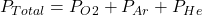## The total pressure of an O2-Ar-He gas mixture is 43 atm. If the partial pressure of Ar is 15 atm and the partial pressure of He is 7 atm, th

Question

The total pressure of an O2-Ar-He gas mixture is 43 atm. If the partial pressure of Ar is 15 atm and the partial pressure of He is 7 atm, then the partial pressure of O2 is –

in progress 0
2 months 2021-07-31T02:50:11+00:00 1 Answers 1 views 0

, The partial pressure of O2 is = 21 atm

Explanation:

The concept is the pressure exerted by each gas is independent of one another or in other words independent of the pressure exerted by all other gases present.

This means that total pressure is the sum of the individual pressure of each gas by dalton’s law.So, putting the given values we get

43 = P_O2 +15+7

P_O2 = 21 atm

Therefore, The partial pressure of O2 is = 21 atm